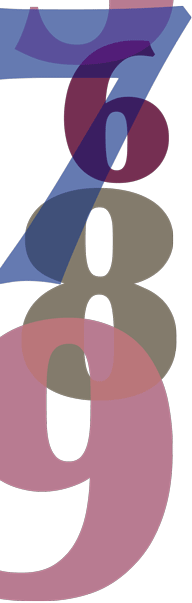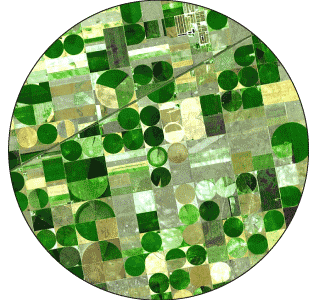# Year 8

## Measurement and Geometry

### Circles

The circle is the most commonly seen figure whose boundary is not a straight line. Coins, plates and tables are often in the shape of a circle, and racetracks generally have semicircular ends. Fans move in circular motion. The circular wheel is widely regarded as one of humanity’s most important inventions, partly because it was integral to cultivation. Many farmers now plant crops in circular fields as this excerpt from a NASA picture of crops in Kansas shows.Source: NASA

When we perform constructions, we use a ruler to draw a line and a compass to draw a circle. Lines and circles are thus fundamental in geometry.

When a wheel is rolled in the sand through one revolution, what length is traced out? This length is the distance around the circle and is called the circumference of the circle. Clearly, the larger the radius, the distance from the centre to the circumference, the greater the circumference of the circle is. Exactly how are the radius and circumference related?

Can we make sense of the area bounded by a circle in the same way that we define the area of a rectangle? Again it seems clear that the larger the radius of the circle is, the larger the area of the circle is. Exactly how are the radius of a circle and the area of a circle related?

Experimental evidence (using string) leads one to see that if the diameter of a circle is doubled, then the circumference is doubled, and more generally that the circumference is proportional to the diameter. This was first investigated by the Greeks who introduced the notion of a proportionality constant between the circumference and the diameter, to which we now give the symbol π (pi). We shall investigate π further in another resource.

We use the term 'area of a circle' to mean the area of the region contained within the circle. Strictly speaking, a circle together with its interior is called a 'disc', but we generally use the word 'circle' to refer both to the circle and the disc.